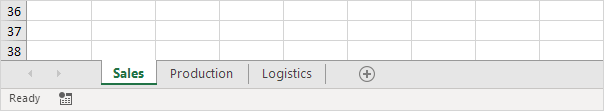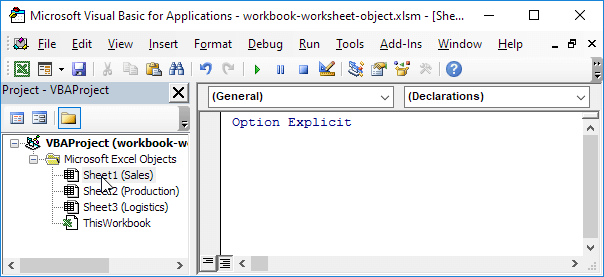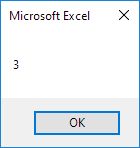# Workbook and Worksheet Object

### Object Hierarchy

In Excel VBA, an object can contain another object, and that object can contain another object, etc. In other words, Excel VBA programming involves working with an object hierarchy. This probably sounds quite confusing, but we will make it clear.

The mother of all objects is Excel itself. We call it the Application object. The application object contains other objects. For example, the Workbook object (Excel file). This can be any workbook you have created. The Workbook object contains other objects, such as the Worksheet object. The Worksheet object contains other objects, such as the Range object.

The Create a Macro chapter illustrates how to run code by clicking on a command button. We used the following code line:

Range("A1").Value = "Hello"

but what we really meant was:

Application.Workbooks("create-a-macro").Worksheets(1).Range("A1").Value = "Hello"

Note: the objects are connected with a dot. Fortunately, we do not have to add a code line this way. That is because we placed our command button in create-a-macro.xlsm, on the first worksheet. Be aware that if you want to change things on different worksheets, you have to include the Worksheet object. Read on.

### Collections

You may have noticed that Workbooks and Worksheets are both plural. That is because they are collections. The Workbooks collection contains all the Workbook objects that are currently open. The Worksheets collection contains all the Worksheet objects in a workbook.You can refer to a member of the collection, for example, a single Worksheet object, in three ways.

1. Using the worksheet name.

Worksheets("Sales").Range("A1").Value = "Hello"

2. Using the index number (1 is the first worksheet starting from the left).

Worksheets(1).Range("A1").Value = "Hello"

3. Using the CodeName.

Sheet1.Range("A1").Value = "Hello"

To see the CodeName of a worksheet, open the Visual Basic Editor. In the Project Explorer, the first name is the CodeName. The second name is the worksheet name (Sales).Note: the CodeName remains the same if you change the worksheet name or the order of your worksheets so this is the safest way to reference a worksheet. Click View, Properties Window to change the CodeName of a worksheet. There is one disadvantage, you cannot use the CodeName if you reference a worksheet in a different workbook.

### Properties and Methods

Now let's take a look at some properties and methods of the Workbooks and Worksheets collection. Properties are something which an collection has (they describe the collection), while methods do something (they perform an action with an collection).

Place a command button on your worksheet and add the code lines:

1. The Add method of the Workbooks collection creates a new workbook.

Note: the Add method of the Worksheets collection creates a new worksheet.

2. The Count property of the Worksheets collection counts the number of worksheets in a workbook.

MsgBox Worksheets.Count

Result when you click the command button on the sheet:Note: the Count property of the Workbooks collection counts the number of active workbooks.

Go to Next Chapter: Range Object